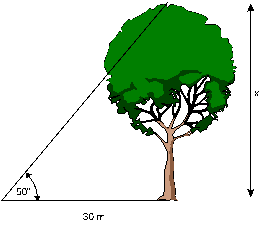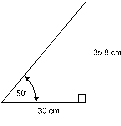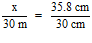# Trigonometry

Trigonometry means “triangle measure”. It is a branch of mathematics which was originally developed to calculate unknown sides and angles in triangles. Trigonometry is very useful in applications such as surveying, navigation and electronics.

### Example 1

Suppose you were a forester and you wanted to know the height of a tree without having to climb the tree with a measuring tape. You can find the height by taking two measurements from the ground (Figure 3).Figure 3Figure 4

Once the distance from the base of the tree is known, as well as the angular measurement to the top of the tree, you can make a scale drawing of a similar triangle and measure the length of the corresponding side (Figure 4).

Using the proportion:the tree is 35.8 m tall.

By using trigonometry, you can solve problems like this in an even simpler fashion.